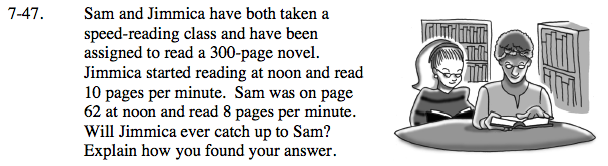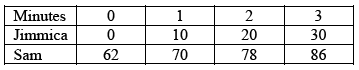### Home > CAAC > Chapter 7 > Lesson 7.1.5 > Problem7-47

7-47.If you are having a hard time getting started, try making a table.Write equations for the situation. Use the table if you need help. Remember to define your variables.

y = the number of pages read
x = the time in minutes

Jimmica: y = 10x
Sam: Write a similar equation.

Solve your system of equations using the Equal Values Method.

Remember - there are only 300 pages in the book. Will Jimmica ever catch up to Sam?